Latest Banking jobs   »

# Quantitative Aptitude Quiz For IDBI AM/Executive 2022- 12th June

Q1. Simple interest for a period of two year is given as Rs. 1800 and the difference between CI and SI for two years is Rs. 270. Find the CI for three years given that sum is same and rate of interest is also same ?
(a) None of these
(b) Rs. 3551
(c) Rs. 3135
(d) Rs. 2721
(e) Rs. 3591

Q2. Sam is 20% less efficient than Anu & both complete the work together in 20 days. If another person Mohit takes 10 days more than Anu and Sam together to complete the same work. Then find in how many days all three complete the work together ?
(a) 10 days
(b) 18 days
(c) None of these
(d) 12 days
(e) 15 days

Q3. Find the ratio of number of words formed from all the letters of ‘ORDINANCE’ when all vowels are together to the number of words formed from all the letters of ‘AMOUNT’?
(a) None of these
(b) 15 : 2
(c) 12 : 1
(d) 16 : 1
(e) 36 : 5

Q4. Deepak made 20% profit by selling his scooter at 25% discount. If the MRP is made two times of previous and offers 40% discount. Then find ratio of previous profit percent to new profit percent?
(a) 1 : 7
(b) 7 : 23
(c) 5 : 21
(d) 5 : 23
(e) None of these

Q5. Ratio of age of Amit and his father 5 years ago is 2 : 5. If age of his mother is 20% more than the present age of his father. Then find difference of age of his father and mother after 10 years given that age of Amit 3 year hence will be 32 years ?
(a) 11 years
(b) 13 years
(c) 8 years
(d) 17 years
(e) 15 years

Directions (6-10): Read the given information carefully and answer the following questions.
Sony Company has five factories with different capacities of producing earphones every month (working days for every month may vary for each factory). In the production process, there are only three types of cost occurs i.e. Raw material cost, Labor cost and Selling cost which are different for different factories. Two units of earphone are produced per day by each factory.
Profit/unit= Selling price-(Raw material cost+ Labor cost+ Selling cost)
Selling cost=Packaging cost+ Transportation cost
Sales Margin= (Profit/unit)/ (Selling price/unit)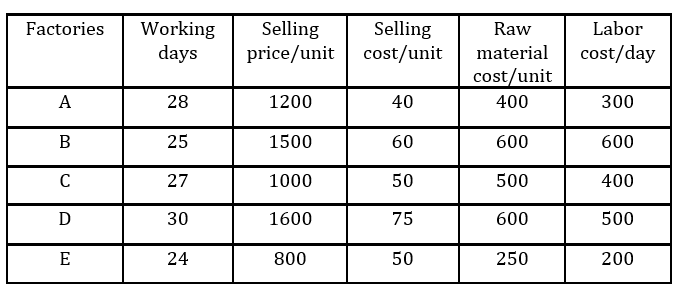Q6. Company used new technology which increased the production by 50% and labor cost by 25% per day for every Factory then find the total profit earned by factories C and D together are approximately what percent more or less than profits earned by Factory A and B together?
(a) 15%
(b) 5%
(c) 10%
(d) 20%
(e) 25%

Q7. A store ordered 80 units of pre-packaged earphone and will also be picked up by that store in 20 days to avoid transportation cost. To deliver this order Company can use multiple factories for production. Find which of the following options will ensure maximum profits for the company?
(a) Factory D, Factory B & Factory A
(b) Factory A & Factory D
(c) Factory A & Factory B
(d) Factory B, Factory D & Factory C
(e) Factory D & Factory E

Q8. For factory B, C, D and E, which of the following order is correct regarding Sales Margin?
(a) D>C>E>B
(b) C >D=B>E
(c) E=D>C>B
(d) E>D>B>C
(e) C>D>E>B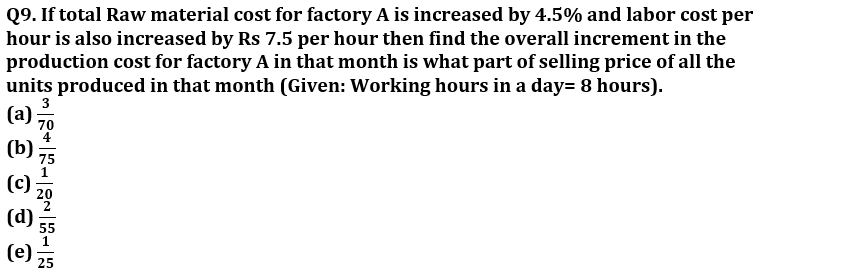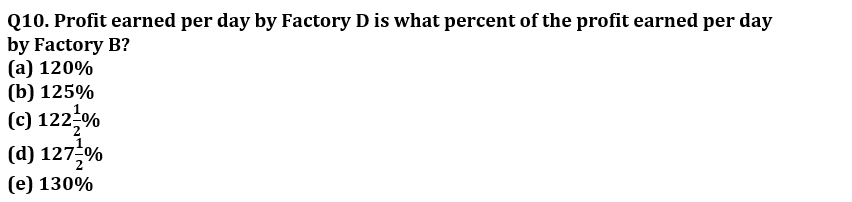Directions (11-15): What will come in the place of question (?) mark.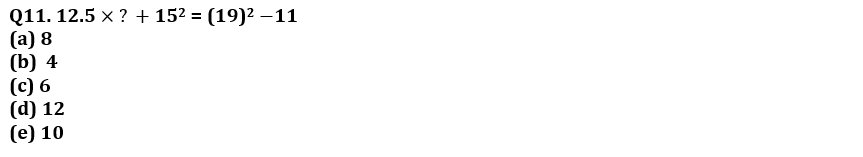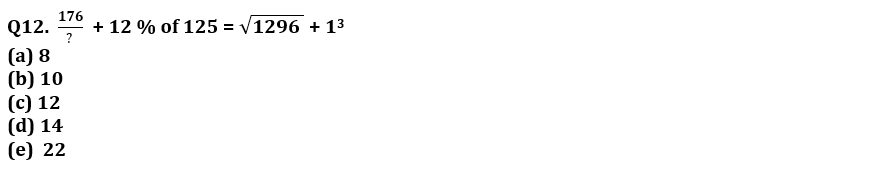Q13. 128 + 15% of ? = (5)³ + 30
(a) 160
(b) 220
(c) 200
(d) 180
(e) 196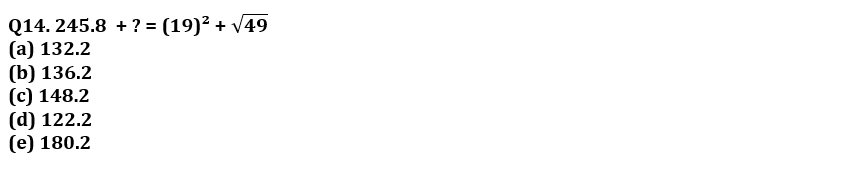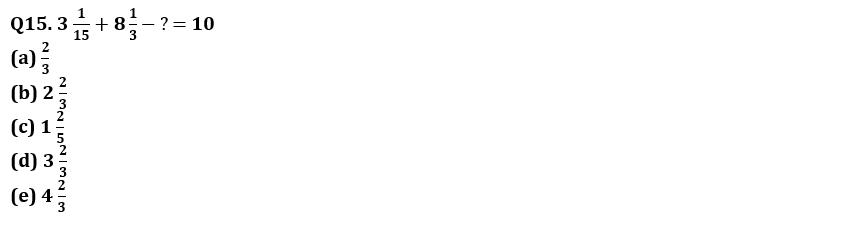Solutions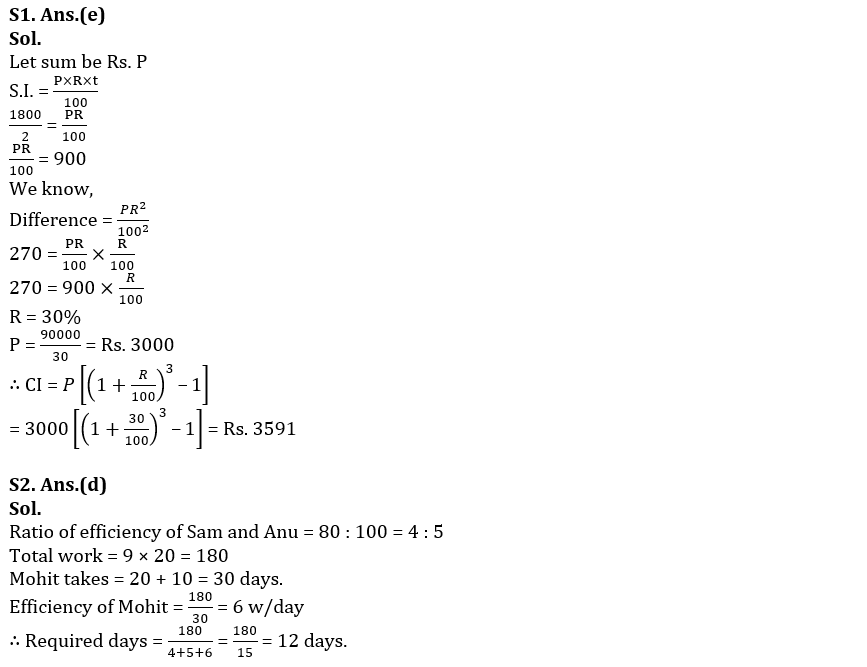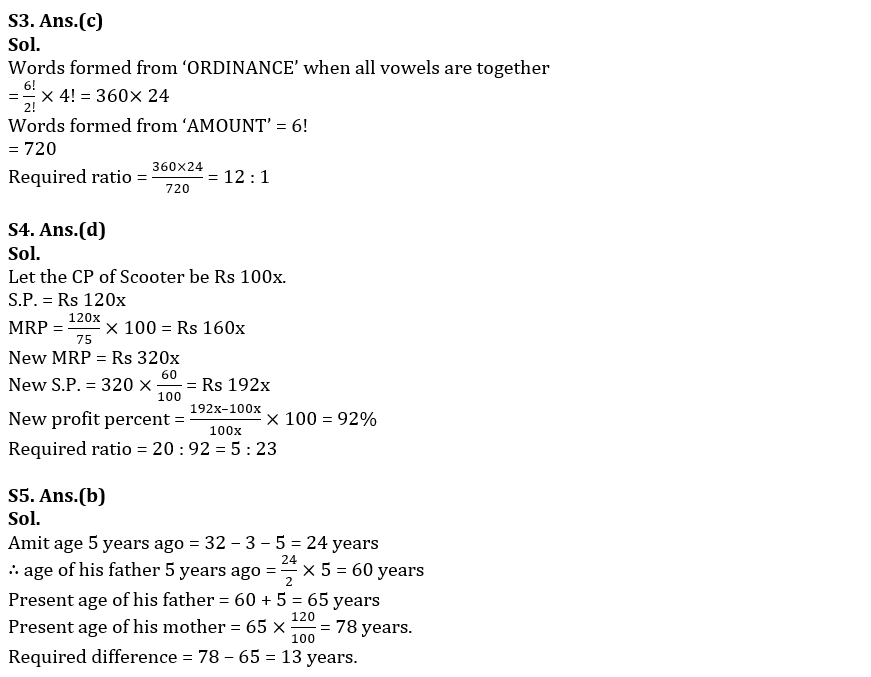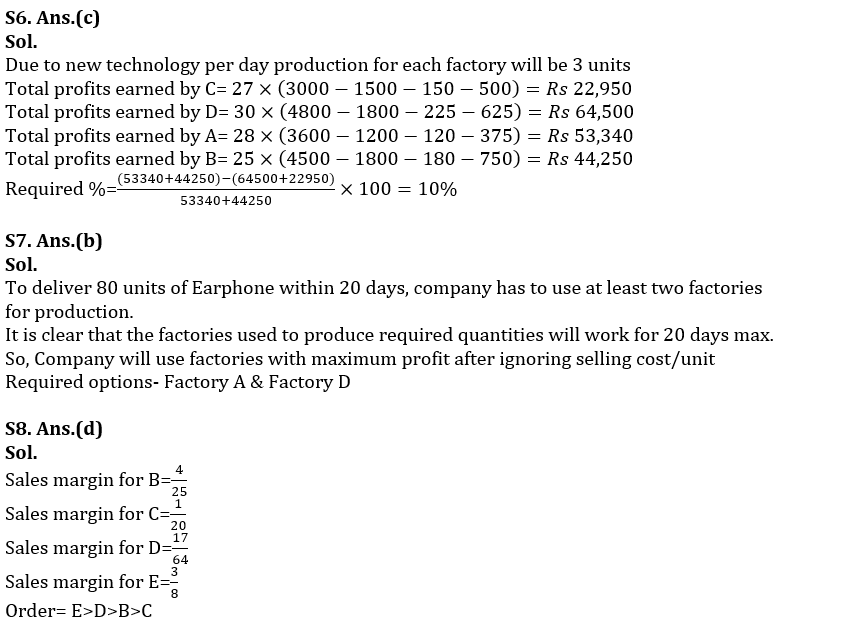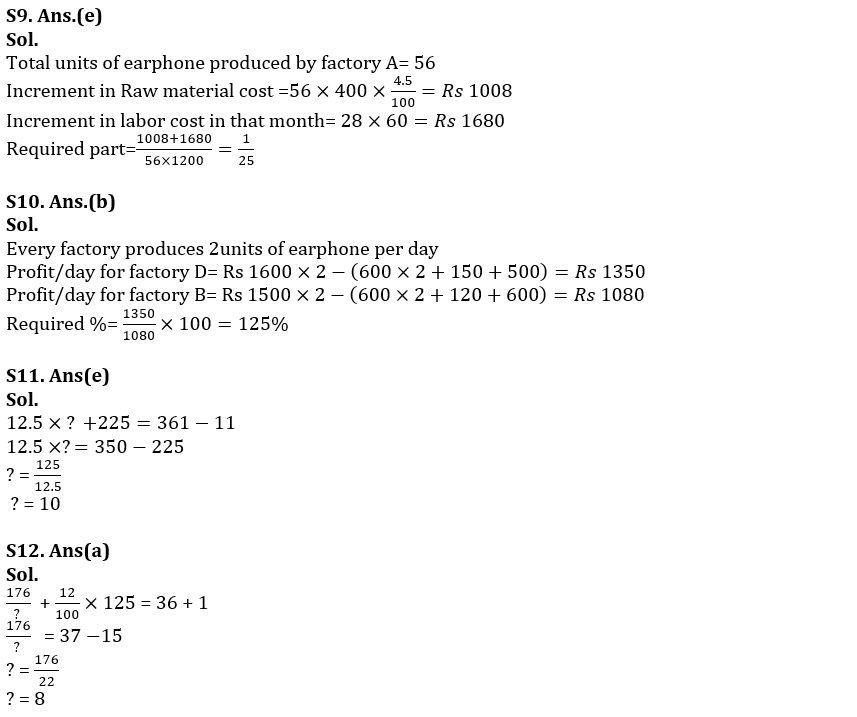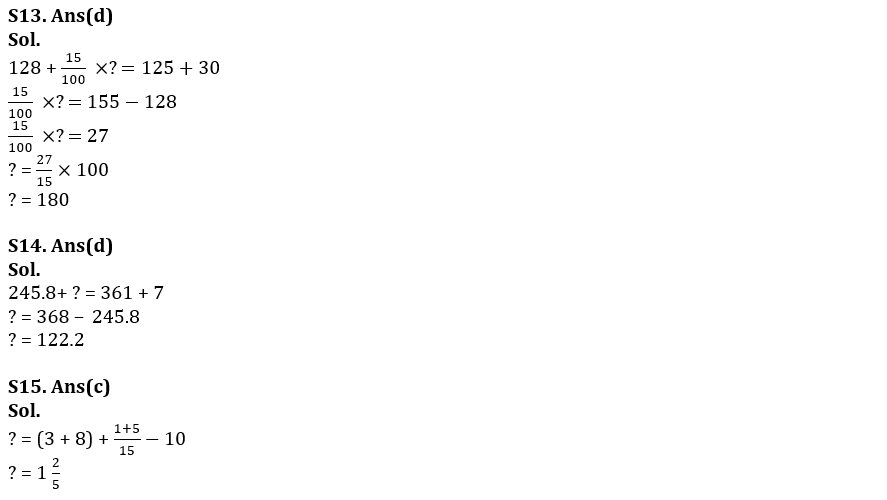#### Congratulations!